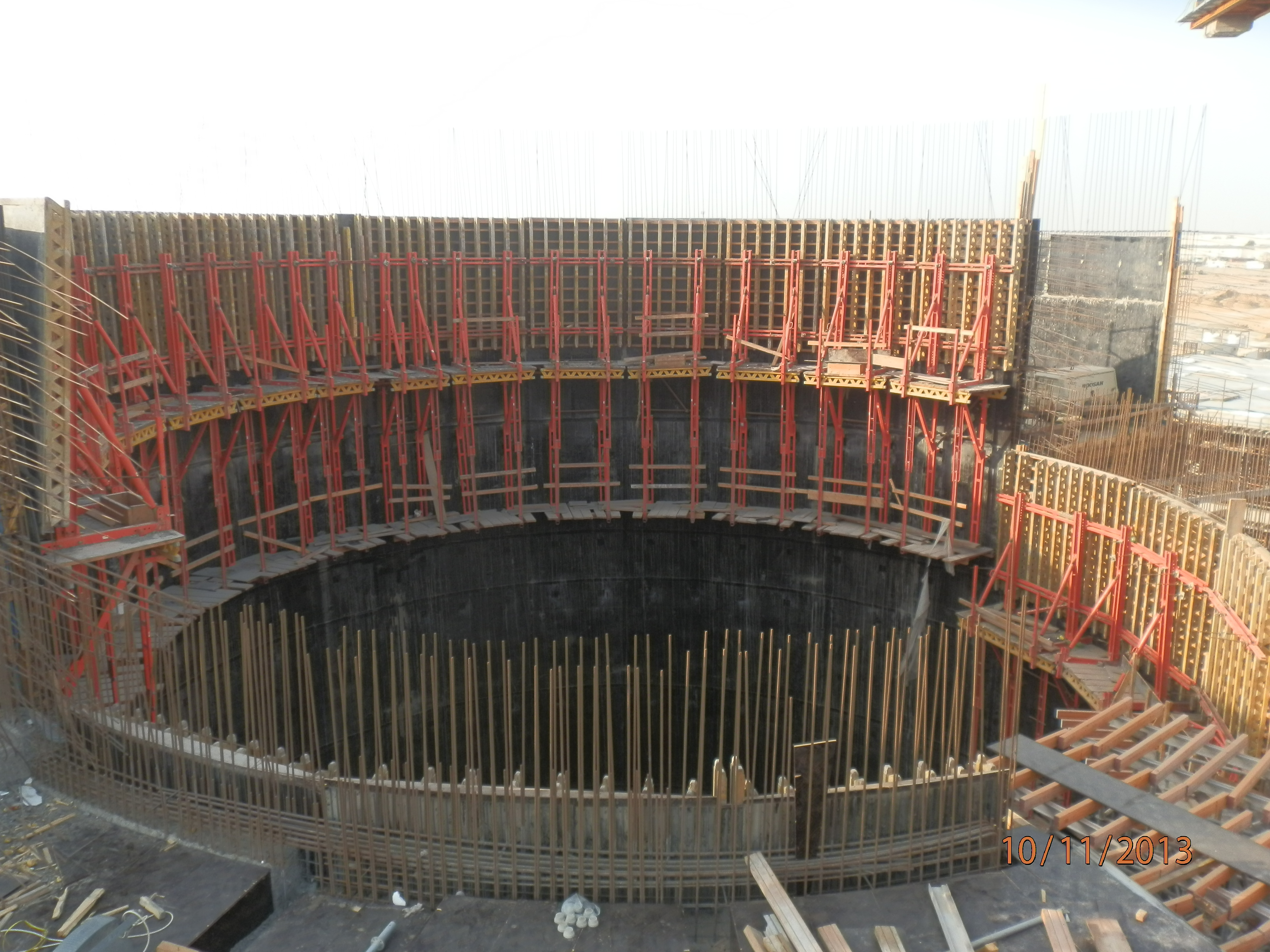# Basic Hydraulic Design CriteriaBasic Hydraulic design criteria:

This article explains the  Hydraulic design criteria and basis for a pumping station:

The pumping station is a hydraulic engineering system used to pump water from a lower water body into a higher one, usually, it consist of three main civil structures which is in most cases the last shaft before the pumping shaft, which we call the screening shaft, because it contains the screens, the grinders and the screw conveyors, these equipment works on racking the sludge out of the water and deliver it to a special housing, and then we have the pumping shafts which contains the pumps, these lift the water to a certain level into a special chamber, we call it the distribution chamber, because from this chamber, the water will be distributed into the related treatment plants, and then we have the facilities and support buildings, such as HVAC building, the generator building and Odour control building. Every aspect of what is considered to be a structural challenge requires detailed design and accurate calculations, therefore considering the minor ratios that can affect your design is vital for a continued successful operation of the station. In this article the focus will be on the Mechanical and process engineering aspects of a pumping station, because as a mechanical engineer, this is the scope of work that I can get more detailed and professional describing, therefore, I will skip the structural and civil engineering part and jump write into the fluid mechanics, which is no less complicated, but I will try to summarize the most important calculations and phases needs to be realized in order to understand how a pumping station works, and maybe later on, more info will be added for the hope to reach a professional understanding of how hydraulics in a pumping station works:

Basic hydraulic calculations needs to be conducted when designing a pumping station:

- Calculating the maximum design, intake, outgoing, flow plus the peak flow in all phases plus the maximum general outfall and the maximum future outfall, the calculating unit shall be in m3/d.

- These data should be compatible In order to preserve consistency in relation to the capacity of the distributing chamber and the related treatment plant, which means studying the treatment plant levels.

Hydraulic formulas to be used:

(1) Gravity sewer-Manning Formula: q = A v = A (kn / n) Rh2/3 S1/2
Manning's equation can be used to calculate cross-sectional average velocity flow in open channels

(2) Weir,Francis formula which define the flow through a rectangular weir, q = 2/3 cd b (2 g)1/2 h3/2
q = flow rate (m3/s)
h = elevation head on the weir (m)
b = width of the weir (m)
g = 9.81 (m/s2) - gravity
cd = discharge constant for the weir - must be determined

(3) calculating roughness factors in channels, GRP pipes and stainless steel pipes, which is usually already have been calculated for these different materials and only need to be taken in to consideration.

(4) The head lose calculations for pressure pipes can occur due to different Friction factors which can be determined on the basis of type of flow i.e. Laminar flow, Transition flow and turbulent flow.

1. Linear head loss for pressure pipes: Head losses are a result of wall friction in all types of pipelines and of local resistance to flow, and it will be calculated with the help of Darcy-Weisbach formula:

hf = f (L/D) x (v2 /2g)

where: hf = head loss (m)

f = friction factor

L = length of pipe work (m)

d = inner diameter of pipe work (m)

v = velocity of fluid (m/s)

g = acceleration due to gravity (m/s²

2. Minor head loss for pressure pipes: which in most cases ought to be measured experimentally , but the common formula used when calculating it is:

hL = ∆p/ρg = KL (v 2/ 2g)

3. Singular head loss for open channel flow: which considers the difference in velocity for a fluid in a channel before and after expansion, and can be calculated with the Borda formula which is a derivation from bernoulli Formula, but it takes in to consideration the empirical loss coefficient ξ

∆H = ξ (V1 – V2)²/ 2g

As mentioned before this is not an attempt to cover as points and study cases as possible but to give a general grasp and hints about how the hydraulics in a pumping station works and what formulas govern its movement.

In the next article, the focus will be on the calculations required for selecting pumps capacity. I wish you all a pleasant reading.